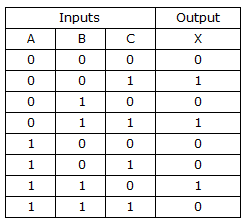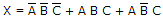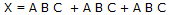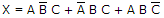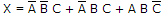# Digital Electronics - Boolean Algebra and Logic Simplification

Exercise : Boolean Algebra and Logic Simplification - General Questions
1.

Convert the following SOP expression to an equivalent POS expression.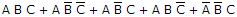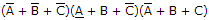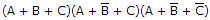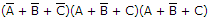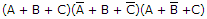Explanation:
No answer description is available. Let's discuss.

2.
Determine the values of A, B, C, and D that make the sum termequal to zero.
A = 1, B = 0, C = 0, D = 0
A = 1, B = 0, C = 1, D = 0
A = 0, B = 1, C = 0, D = 0
A = 1, B = 0, C = 1, D = 1
Explanation:
No answer description is available. Let's discuss.

3.
Which of the following expressions is in the sum-of-products (SOP) form?
(A + B)(C + D)
(A)B(CD)
AB(CD)
AB + CD
Explanation:
No answer description is available. Let's discuss.

4.

Derive the Boolean expression for the logic circuit shown below: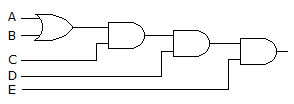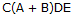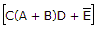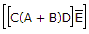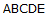Explanation:
No answer description is available. Let's discuss.

5.

From the truth table below, determine the standard SOP expression.